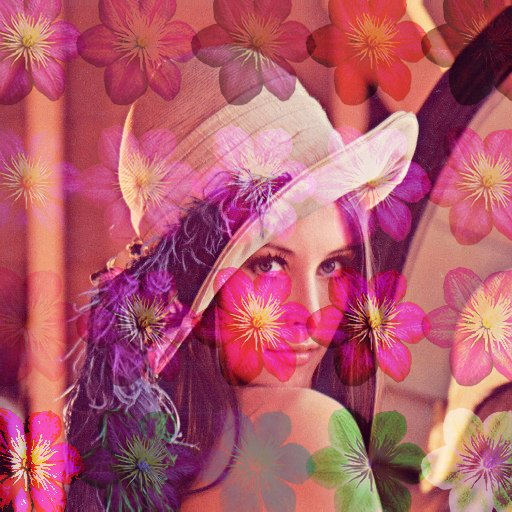## PPMage

phpimage processinglibrary

PPMage — lightweight PHP library for applying effects to images.

## Usage

``````include_once('ppmage/PPMage.php');
\$image = PPMage::fromFile('source.jpg')->
// Applying effects
eInvert(1, 1, 1, 0)->
eBoxBlur(5, 5)->
eExtractChannel(2, 0)->
eInvert(0, 1, 0, 0)->
// Getting result image
getImage();
// Saving to file
imagejpeg(\$image->getData(), 'result.jpg', 85);
// Or show as base64
echo '<img src="data:image/png;base64,', \$image->exportAsBase64(), '" alt="base64_image" />';
// Retrieving an image information
echo '<br/>Size: ', \$image->getWidth(), 'x', \$image->getHeight();
echo '<br/>Type: ', \$image->getType();
``````
• File:
``PPMage::fromFile("file.jpg")``
• URL:
• ``PPMage::fromUrl('http://lorempixel.com/400/200/')``
• From image data:
• ``````\$image = imagecreatetruecolor(240, 50);
PPMage::fromParameters(240, 50, \$image);``````

## Effects

Source image### Decolour

Converts image to grayscale.
``eDecolour()``### Inverting channels

Inverts one or more selected channel.
`eInvert(red [0..1], green [0..1], blue [0..1], opacity [0..1])`
``````// Inverts blue channel
eInvert(0, 0, 1, 0)
``````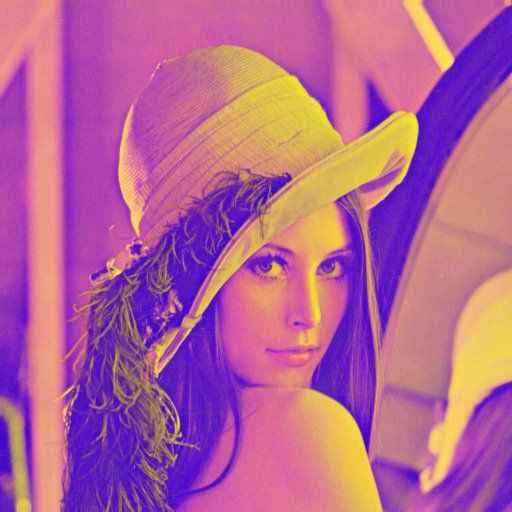``````// Inverts all channels (negative)
eInvert(1, 1, 1) // parameters are optional
``````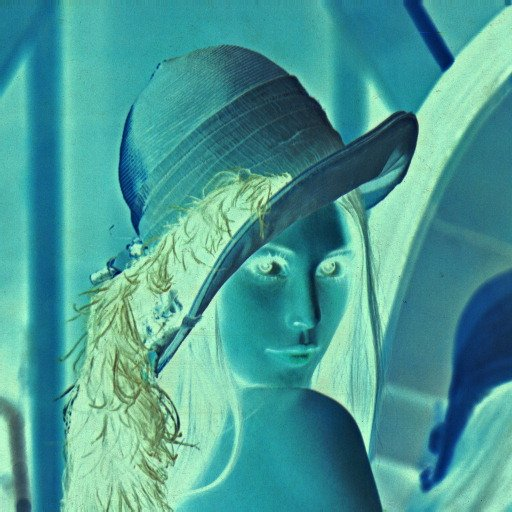### Solarize

Solarize effect.
``````eSolarize()
``````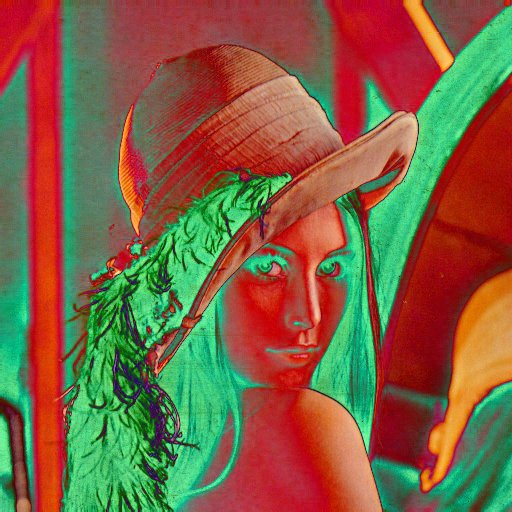### Posterization

ПApplies the effect of posterization (coarsening).

`ePosterization(value [0..255])`
The higher the value, the rougher the colors.
``````ePosterization(64)
``````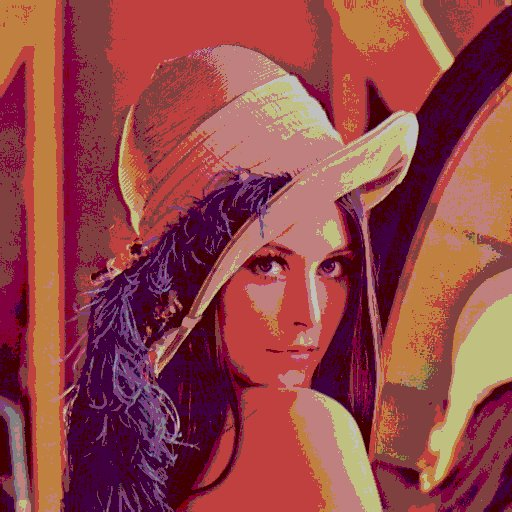``````ePosterization(200)
``````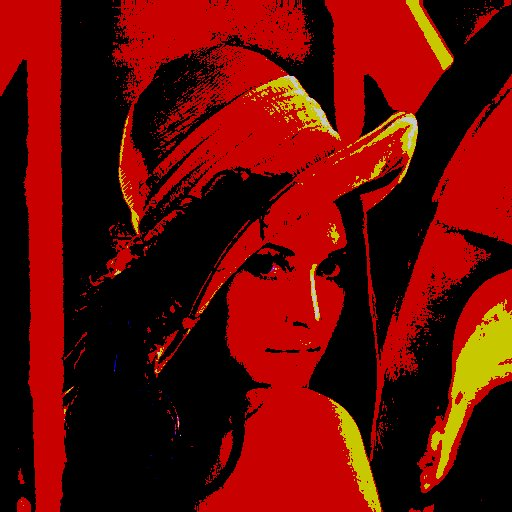### Extract channel

Extracts one channel from image (opacity, red, green, blue). It can also convert this channel to grayscale.
NOTE: for opaque images, extracting a transparent channel returns a black image. `eExtractChannel(channel [0..3], grayscale [0..1])`
• Channel:
• 0 - opacity
• 1 - red
• 2 - green
• 3 - blue
``````// extracts red channel
eExtractChannel(1, 0)
``````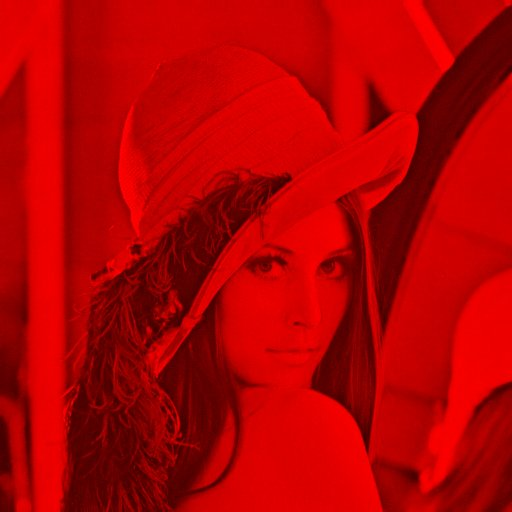``````// extracts green channel
eExtractChannel(2) // you can omit the default parameters
``````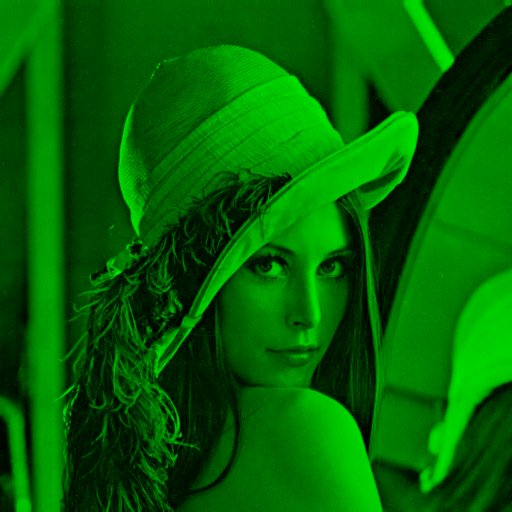``````// extracts blue channel, converting it to grayscale
eExtractChannel(3, 1)
``````### RGB-correction

Changes red, green, blue channel and opacity.

`eRGBCorrection(red [-255..255], green [-255..255], blue [-255..255], opacity [-255..255])` NOTE: when changing the transparency, you should save the image in png or gif format, otherwise this channel is ignored.
``````eRGBCorrection(-70, -18, -221)
``````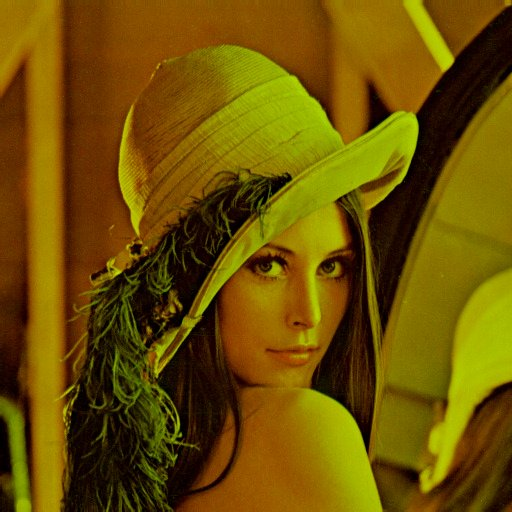``````// RGB correction with changeing transparency
eRGBCorrection(-255, -140, 217, -180)
``````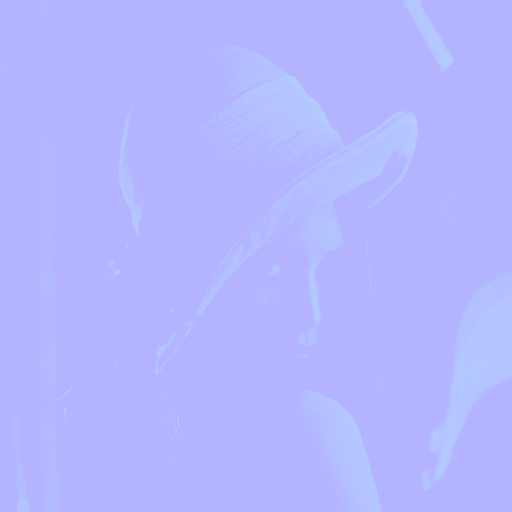### HSB-correction

Changes the hue, saturation, and brightness of the image.

`eHSBCorrection(hue [0..360], saturation [-100..100], brightness [-100..100], tinting [0..1])`

If Tinting> mode is enabled, the image will be colored in the specified hue, otherwise the value of the Hue parameter will be added to each color.

``````// Change the hue by 259 degrees,
// and decrease the brightness by 5
eHSBCorrection(259, 10, -5, 0)
``````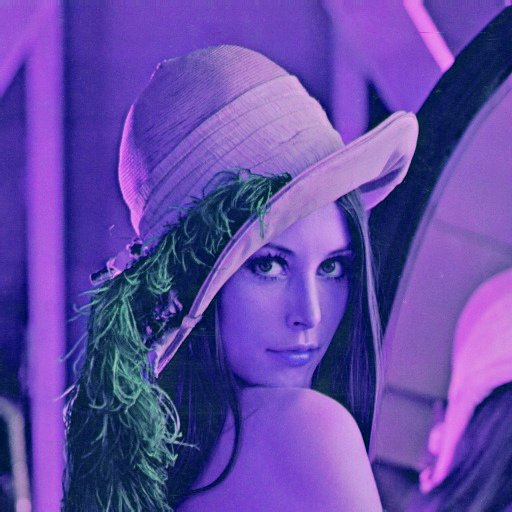``````// Image tinting
eHSBCorrection(259, 10, -5, 1)
``````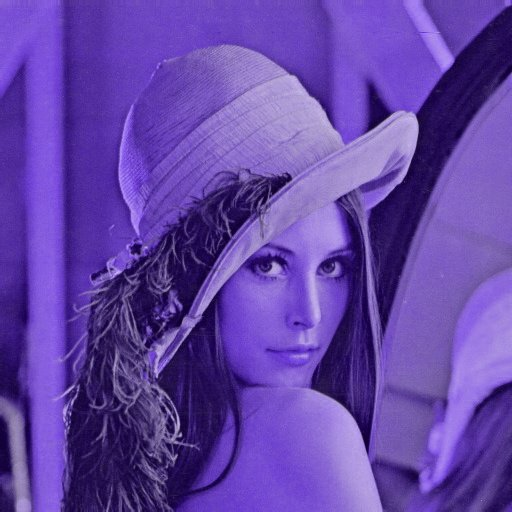### Saturation

Changes the saturation of the image.

`eSaturation(value [-255..255])`
``````// Decreasing saturation
eSaturation(-164)
````````````// Increasing saturation
eSaturation(100)
``````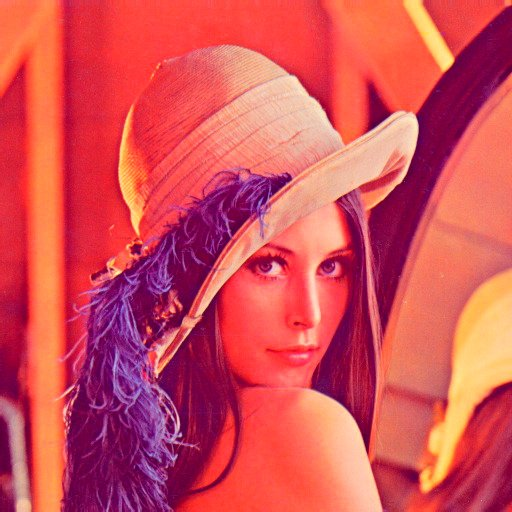### Contrast

Changes the contrast of the image.

`eContrast(value [-100..100])`
``````// Decreasing contrast
eContrast(-80)
````````````// Increasing contrast
eContrast(60)
``````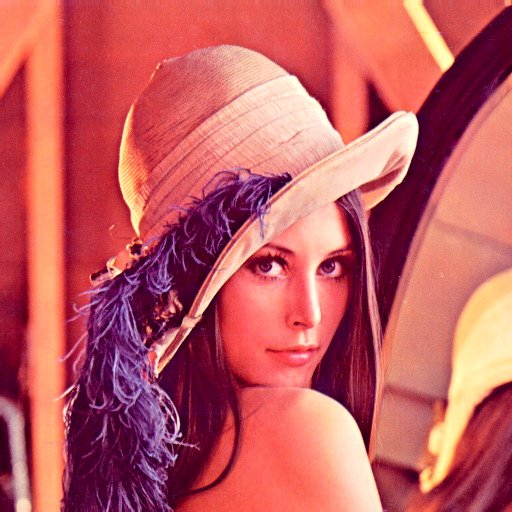### Gamma

Changes the gamma level of the image.

`eGamma(value [-50..50])`
``````// Gamma decreasing
eGamma(-40)
````````````// Gamma increasing
eGamma(25)
``````### Blur

Applies a blur effect.

`eSmooth(value [1..25])` NOTE: the larger the value, the longer the effect is applied.
``````eSmooth(6)
``````### Box blur

Uses a fast box blur in two directions.

`eBoxBlur(horizontal [1..100], vertical [1..100])`
``````// Horizonal blur
eBoxBlur(100, 1)
``````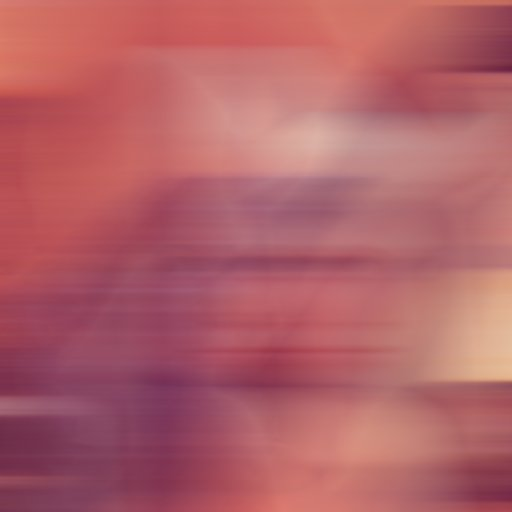``````// Vertical blur
eBoxBlur(1, 100)
``````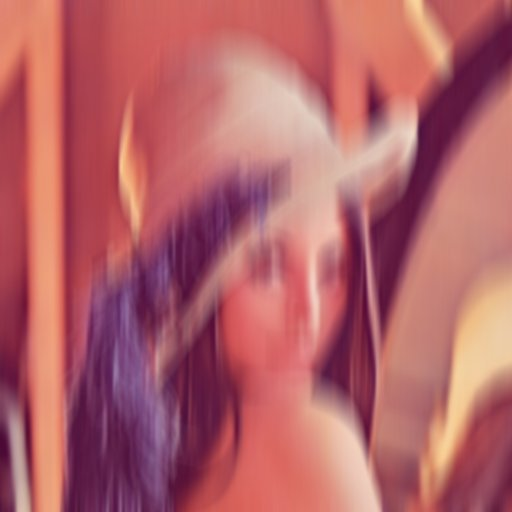``````// Two directional blur
eBoxBlur(20, 20)
``````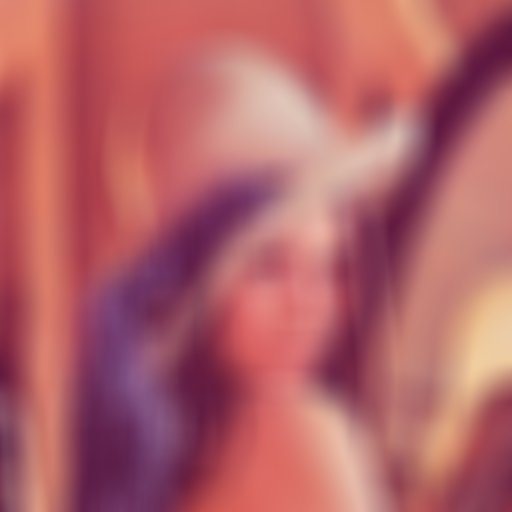### Edge detection

Applies the edge detection effect for four well-known operators (Roberts, Prewitt, Sobel, and Scharr). You can also get the result as colored edges, grayscale edges, or as an overlay of edges on the original image.

`eEdgeDetection(operator [0..3], mode [0..2])`
• Operator:
• 0 - Roberts
• 1 - Prewitt
• 2 - Sobel
• 3 - Scharr
• Mode:
• Colored edges
• Grayscale edges
• Edges subtraction
``````// Roberts operator and grayscale edges
eEdgeDetection(0, 1)
``````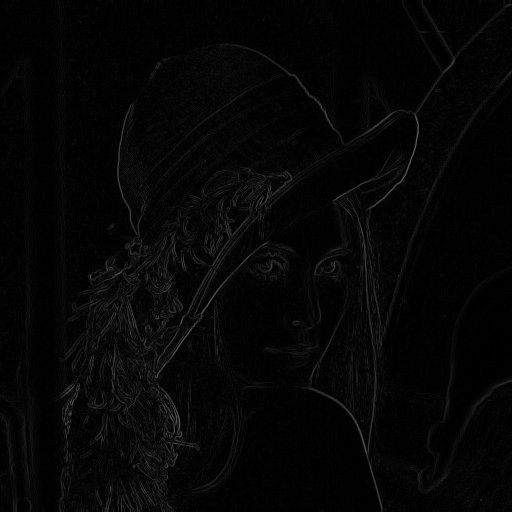``````// Prewitt operator and colored edges
eEdgeDetection(1, 0)
``````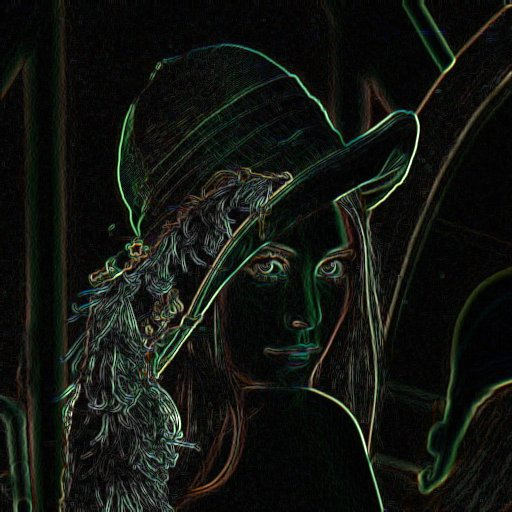``````// Sobel operator and egdes subtraction
eEdgeDetection(2, 2)
``````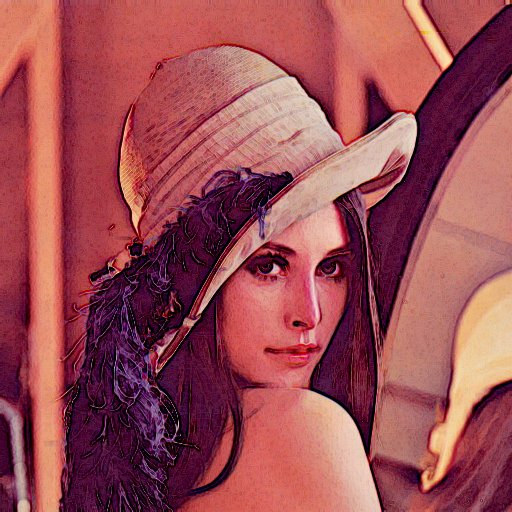``````// Scharr operator and grayscale edges
eEdgeDetection(3, 1)
``````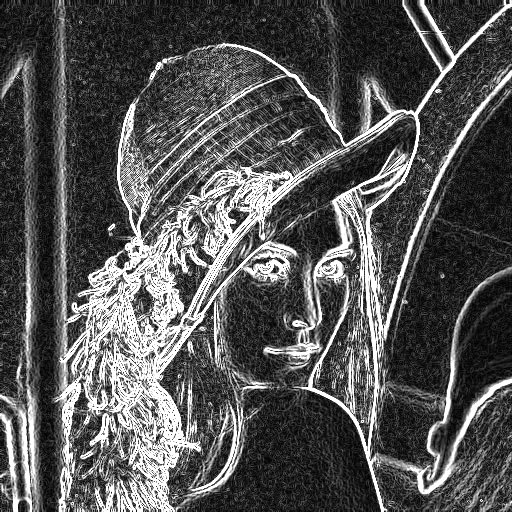### Scatter

Applies the effect of the scatter of pixels in two directions.

`eScatter(horizontal [1..100], vertical [1..100])`
``````// Horizontal scatter
eScatter(40, 1)
``````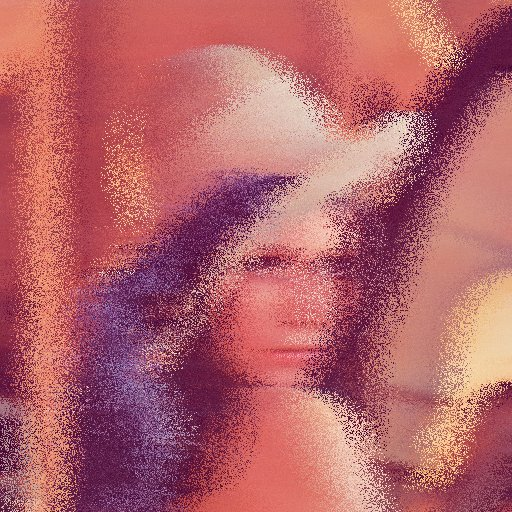``````// Vertical scatter
eScatter(1, 80)
``````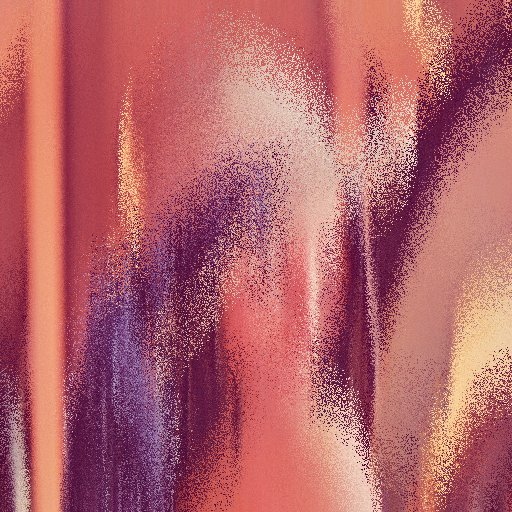``````// Two-directional scatter
eScatter(20, 20)
``````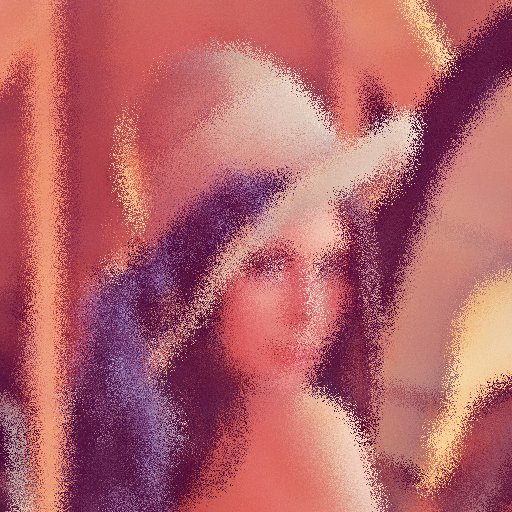### Noise

Overlays the image with noise-colored or black-and-white dots.

`eNoise(overlaying [1..255], grayscale [0..1])`
``````// Overlay of colored noise
eNoise(100, 0)
``````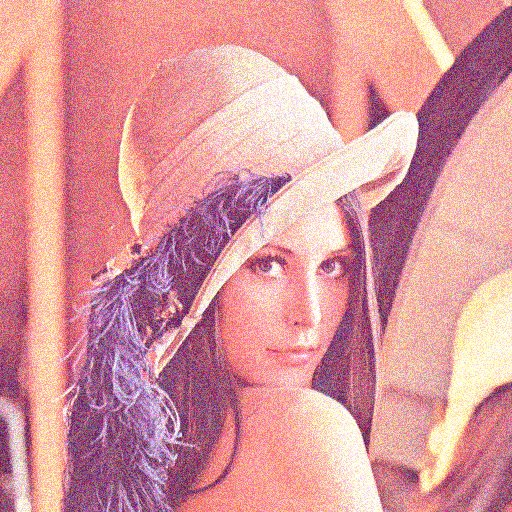``````// Overlay of grayscale noise
eNoise(150, 1)
``````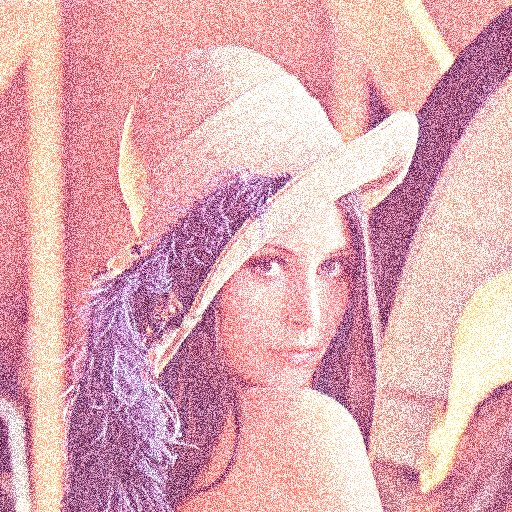### Convolution

Applies a convolution matrix to the image. The effect is similar to imageconvolution function, but it is not limited in the size of the matrix. Due to this, you can get a variety of effects.

`eConvolution(matrix width, matrix height, matrix (array), divisor, offset, border tracking mode [0..1])`
• The matrix is set as an 1d array, not 2d array:
``````eConvolution(5, 3, [
0, 0, 1, 0, 0,
1, 2,-8, 2, 1,
0, 0, 1, 0, 0], ...);
``````
• If the divisor is set to zero, it is automatically calculated as the sum of the matrix values.
• Offset-adds the specified value to each color component. Used when you need to normalize values after applying the convolution kernel.
• Border tracking mode:
• 0 — value 0 will be taken at the border positions;
• 1 — the value of the current pixel will be taken at the boundary values.
``````// Sharpness
eConvolution(3, 3, [
0, -1, 0,
-1,  5, -1,
0, -1, 0], 0, 0, 1)
````````````// Soft sharpness (float numbers are used).
eConvolution(3, 3, [
-1.2, -1, -1.2,
-1.0, 20, -1.0,
-1.2, -1, -1.2], 0, 0, 1)
````````````// Blur 5x5
eConvolution(5, 5, [
1,  4,  7,  4, 1,
4, 16, 26, 16, 4,
7, 26, 41, 26, 7,
4, 16, 26, 16, 4,
1,  4,  7,  4, 1])
````````````// Emboss
eConvolution(3, 3, [
0, -1, 0,
1,  4, -1,
0, -1, 0], 0, 127, 1)
``````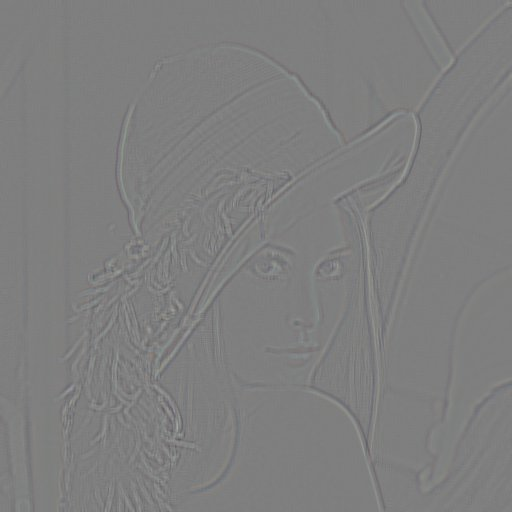### The overlay of two images

Overlay one image on another with different modes (as in Photoshop).

`eImageBlend(image, x, y, overlay mode [0..19], opacity [0..255])`
• x, y — coordinates where the image will be applied. They can be negative if only part of the image needs to be overlaid.
• The overlay modes are sorted in the same order as in Photoshop:
• Normal
• 0 - NORMAL
• Darkening group
• 1 - DARKEN
• 2 - MULTIPLY
• 3 - COLOR_BURN
• 4 - LINEAR_BURN
• Lightening group
• 5 - LIGHTEN
• 6 - SCREEN
• 7 - COLOR_DODGE
• 8 - LINEAR_DODGE
• Contrast enhancement group
• 9 - OVERLAY
• 10 - SOFT_LIGHT
• 11 - HARD_LIGHT
• 12 - VIVID_LIGHT
• 13 - LINEAR_LIGHT
• 14 - PIN_LIGHT
• 15 - HARD_MIX
• Comparison group
• 16 - DIFFERENCE
• 17 - EXCLUSION
• 18 - SUBTRACT
• 19 - DIVIDE
• Transparency is an optional parameter, set to 255 by default. Analogous to the Neopacity parameter in Photoshop, only it is not measured as a percentage, but in numbers from 0 to 255.
``````// Overlay a flower.png image over the entire area
// of the \$source image with different overlay modes
\$flower = PPMage::fromFile('flower.png')->getImage();
\$source = PPMage::fromFile('lena.jpg');
\$srcW = \$source->getImage()->getWidth();
\$srcH = \$source->getImage()->getHeight();
\$stepY = \$flower->getHeight() * 1.1;
\$stepX = \$flower->getWidth() * 0.9;
\$count = 0;
for (\$y = -20; \$y < \$srcH; \$y += \$stepY) {
for (\$x = -40; \$x < \$srcW; \$x += \$stepX) {
\$source = \$source->eImageBlend(\$flower, \$x, \$y, \$count % 20, 128);
\$count++;
}
}
\$image = \$source->getImage();
``````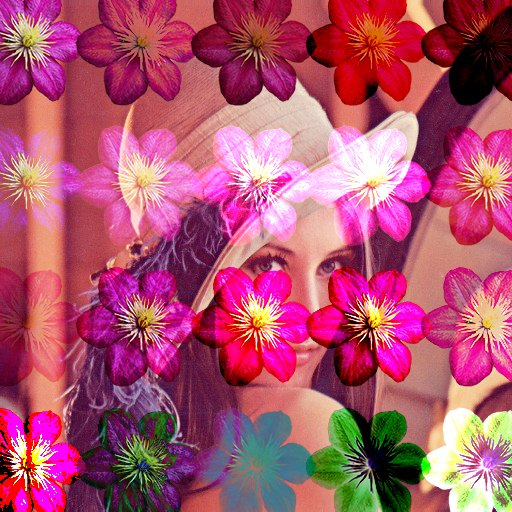``````// Overlay flower.png with opacity 128
\$flower = // ...
// similarly...
\$source = \$source->eImageBlend(\$flower, \$x, \$y, \$count % 20, 128);
\$image = \$source->getImage();
``````